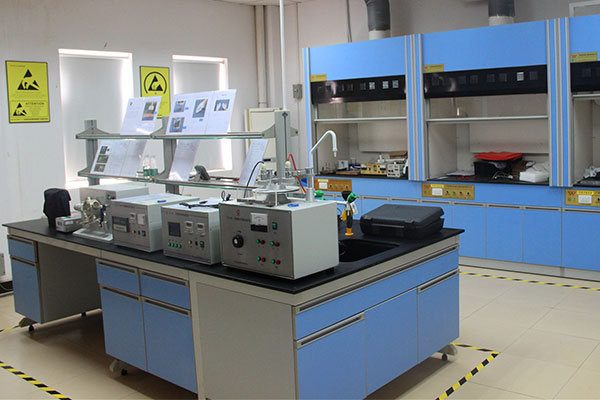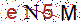#×......

## 防静电检测技术指标

 防静电产品 测试项目 接地电阻（Ω） 表面电阻（Ω） 体电阻（Ω） 系统电阻（Ω） 摩擦电压（V） 防静电地极 接地母线与大地之间：＜4 - - - - 仪器设备、装备、工具 与接地母线之间：硬接地＜1；软接地1×10^6≤Rg＜1×10^9 - - - - 防静电地板 与接地母线之间：0.1×10^6≤Rg＜1×10^9 0.1×10^6≤Rs＜1×10^9 - - ＜100 防静电烙铁头 与接地母线之间：要求＜1可接受＜20 - - - - 腕带 人体腕带接地电阻： 0.75×10^6≤Rg≤10×10^6 - - 腕带内表面对电缆扣电阻： ≤20K；腕带外表面对电缆扣电阻： >1×10^7 ；腕带连接电缆电阻：>0.8 ×10^6 ~<1.2  ×10^6 - 工鞋、鞋跟 0.75×10^6≤R≤100×10^6 - 0.5×10^6≤Rv≤50×10^6 0.75×10^6≤R≤100×10^6 ＜100 台垫、地垫 0.75×10^6≤Rv＜1×10^9 1×10^6≤Rs＜1×10^9(正面)1×10^3≤Rs＜1×10^5(反面) 1×10^6≤Rv＜1×10^9 - ＜100 工衣、工帽、手套 - 0.1×10^6≤Rs＜1×10^10(点与点、袖对袖、片与片) - - ＜100 工作椅 - ＜1×10^9 ＜1×10^9 ＜1×10^9（椅面对脚轮、椅面对靠背） ＜100 防静电周转箱、托盘、器件盒 - 1×10^3≤Rs＜1×10^9 1×10^3≤Rv＜1×10^9 - ＜100 防静电周转车 - 1×10^3≤Rs＜1×10^9（导轨槽） - 1×10^6≤Rs＜1×10^9 ＜100 防静电传送带 0.75×10^6≤Rg＜1×10^9 1×10^6≤Rs＜1×10^9 1×10^6≤Rv＜1×10^9 - ＜100 屏蔽袋 - ＜1×10^11 ＜1×10^11 - ＜100（屏蔽残余电压＜100V） 导电材料 - ＜1×10^4 ＜1×10^4 - ＜100 耗散材料 - 1×10^4 ≤Rs＜1×10^11 1×10^4≤Rs＜1×10^11 - ＜100 绝缘材料 - ≥1×10^11 ≥1×10^11 - - 离子风机 消电时间T1000→100：<2s（高速）（距离风扇1ft处）<3s（低速）离子平衡度：≤±15V或参照仪器说明书 显示器 静电感应电压＜100V 防静电液（抗静电剂） 防静电液均匀涂覆绝缘材料表面后其表面电阻应满足：1×106Ω≤Rs＜1×109Ω。 其它（防静电毛刷、镊子、吸笔等） 防静电参数指标与耗散材料同×

*公司名称

*您的姓名

*您的手机

*您的需求

## 在线留言刷新